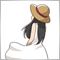# How to know if the number is 4,8,12,16----393

Hi, I want to know if the value is 4 ,8,12,16 .... 4 times XXXXX

For example, I have 13.

```double N=13;

if (N/4)<point{

Alert("It is 4 times value");

}

```

Can you help?820

```if (N % 4 == 0){
Alert("It is 4 times value");
}```
If the remainder of N divided by 4 is 0, then it is a multiple of 4.393

Thank you! but what does this mean?

`N % 4 `820

Cromo:

Thank you! but what does this mean?

% is a arithmetic symbol to find the remainder of the division.

Same as

`MathMod(N, 4)`24704

Cromo: but what does this mean?

Module division is the remainder from the division (A - int(A/B)*B).393

Nagisa Unada:

% is a arithmetic symbol to find the remainder of the division.

Same as

Wow, I have learned a new code.

Thank you very much!393

William Roeder:

Module division is the remainder from the division (A - int(A/B)*B).

Thank you!